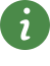# ParameterDefaults

Parameter defaultsUsersGuide

## Description

In this section the convention is summarized how default parameters are handled in the Modelica Standard Library (since version 3.0).

Many models in this library have parameter declarations to define constants of a model that might be changed before simulation starts. Example:

model SpringDamper
parameter Real c(final unit="N.m/rad")    = 1e5 "Spring constant";
parameter Real d(final unit="N.m.s/rad")  = 0   "Damping constant";
parameter Modelica.SIunits.Angle phi_rel0 = 0   "Unstretched spring angle";
...
end SpringDamper;


In Modelica it is possible to define a default value of a parameter in the parameter declaration. In the example above, this is performed for all parameters. Providing default values for all parameters can lead to errors that are difficult to detect, since a modeler may have forgotten to provide a meaningful value (the model simulates but gives wrong results due to wrong parameter values). In general the following basic situations are present:

1. The parameter value could be anything (e.g., a spring constant or a resistance value) and therefore the user should provide a value in all cases. A Modelica translator should warn, if no value is provided.
2. The parameter value is not changed in > 95 % of the cases (e.g., initialization or visualization parameters, or parameter phi_rel0 in the example above). In this case a default parameter value should be provided, in order that the model or function can be conveniently used by a modeler.
3. A modeler would like to quickly utilize a model, e.g.,
• to automatically check that the model still translates and/or simulates (after some changes in the library),
• to make a quick demo of a library by drag-and-drop of components,
• to implement a simple test model in order to get a better understanding of the desired component.
In all these cases, it would be not practical, if the modeler would have to provide explicit values for all parameters first.

To handle the conflicting goals of (1) and (3), the Modelica Standard Library uses two approaches to define default parameters, as demonstrated with the following example:

model SpringDamper
parameter Real c(final unit="N.m/rad"  , start=1e5) "Spring constant";
parameter Real d(final unit="N.m.s/rad", start=  0) "Damping constant";
parameter Modelica.SIunits.Angle phi_rel0 = 0       "Unstretched spring angle";
...
end SpringDamper;

SpringDamper sp1;              // warning for "c" and "d"
SpringDamper sp2(c=1e4, d=0);  // fine, no warning


Both definition forms, using a "start" value (for "c" and "d") and providing a declaration equation (for "phi_rel0"), are valid Modelica and define the value of the parameter. By convention, it is expected that Modelica translators will trigger a warning message for parameters that are not defined by a declaration equation, by a modifier equation or in an initial equation/algorithm section. A Modelica translator might have options to change this behavior, especially, that no messages are printed in such cases and/or that an error is triggered instead of a warning.